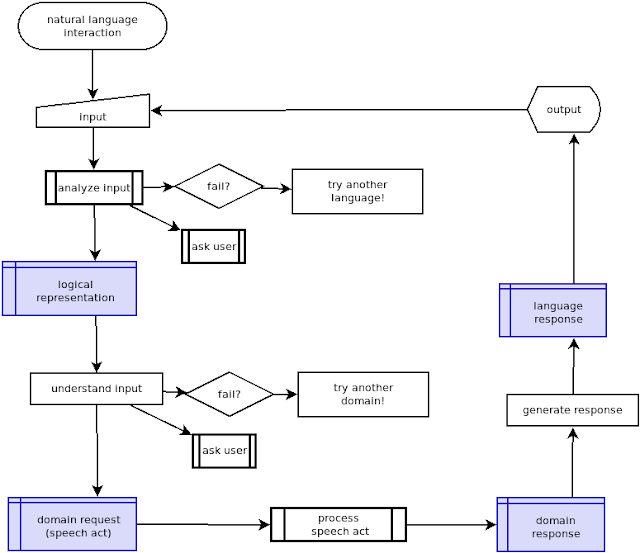zondag 2 december 2018

Matching brackets

For my work as a developer, I sometimes get the chance to interview new developers for the company. I check their resumes and I review some of their code they send me. We thought it would also be a good idea to give them a little programming task to do in a limited amount of time. I had no idea what kind of task to choose, so I checked Google. The following problem showed up several times and I liked it because it requires some thinking to do it right and at the same time, it doesn't require a lot of typing.

Write a function that checks if the parentheses of an expression match.

The task involves several types of brackets: [], () and {}.  For example, the parenthesis in this expression match:
`({}{(56)})`
While in this expression they do not:
`{[909)`
I wrote a test function (in PHP):
```function test(string \$exp, bool \$expected)
{
if (matchBrackets(\$exp) !== \$expected) {
echo "Error matching " . \$exp . "\n";
}
}```
and some tests
```test("()", true);
test("()()", true);
test("noot()noot", true);
test("(aap)", true);
test("aap", true);
test("(", false);
test(")", false);
test("{[]}", true);
test("{[][]}", true);
test("{[]()}", true);
test("", true);
test("{[aap](({banaan}))}", true);
test("{[{[](({}){a[](({}))}){b[](({})){[](({c}))}}{[](({}))}}]({[]d(({}){[](({123}))})}({{[](({}))}}))}", true);
test("{[aap](({banaa))}", false);
test("{[aap]((anaan}))}", false);
```

Solving the problem in an elegant way requires you to use recursive nature of the domain.

While the developer was writing the program, I tried to figure it out for myself. And it occurred to me that next to some algorithmic options, there would also be a possibility to use a regular expression. But this could only be done by applying the advanced recursive patterns feature. I had heard of this feature, but never used it.

I would certainly not demand of the developer to give such an answer, but it intrigued me too much to let it go.

It is indeed possible, and so, without further ado, I present the answer here:
```function matchBrackets(string \$exp): bool
{
return preg_match('/^
# start of group 1
(
(
(
[^\(\{\[\)\}\]]+
) |
(
\( (?1) \)
) |
(
\[ (?1) \]
) |
(
\{ (?1) \}
)
)*
)
\$/x', \$exp);
}
```

I will try to explain by building the expression from the ground up.
`//x`
The "x" modifier strips all whitespace from the string. This allows you to make it more readable.
`/^\$/x`
Match the beginning and end of \$exp.
`/^(recursion-group)\$/x`
Create the group that will be used for recursion.
`/^((recursion-group)*)\$/x`
Each item in this group can be repeated any number of times. This also allows for the completely empty \$exp.
`/^(((other-chars)|(round-brackets)|(squared-brackets)|(curly-braces))*)\$/x`
Any nested group consists of a sequence of non-brackets, or a set of round brackets () or a set of squared brackets [] or a set of curly braces {}. Finally a "bracket" groups look like this:
`\{ (?1) \}`
It starts with a bracket (here "{"), followed by a recursive group, and ends with the matching bracket "}"..

A recursive group can repeat the entire expression. You would have to use (?R) in stead of (?1). Here it just matches a single group (group 1) from the expression. Group 1 is the group that starts with the first "(" in the expression. I do not use (?R) here because that would place the ^ and \$ in the middle of the expression. That would never give a match.

End Note

It took me hours to get this expression just right, and as simple as possible. I expected the recursive expression to be complicated, but actually it turned out that it could be made to be pretty simple. And if you're interested: yes, it is fast. It was about 8 times as fast as the algorithmic variant I came up with. For a non-compiled language like PHP regular expressions open the door to the speed of a compiled language.

Natural language interaction in flowcharts

Flowchart In this post I will sketch some of my ideas about a generic natural language interaction program in the form of a flowchart. N...